# Integrating Biot-Savart Law at a point

• ChiralSuperfields
ChiralSuperfields
Homework Statement
Relevant Equations
Why when you integrate the Biot-Savart Law do we not include limits of integration on the left-hand side of the equation (for the differential magnetic field)?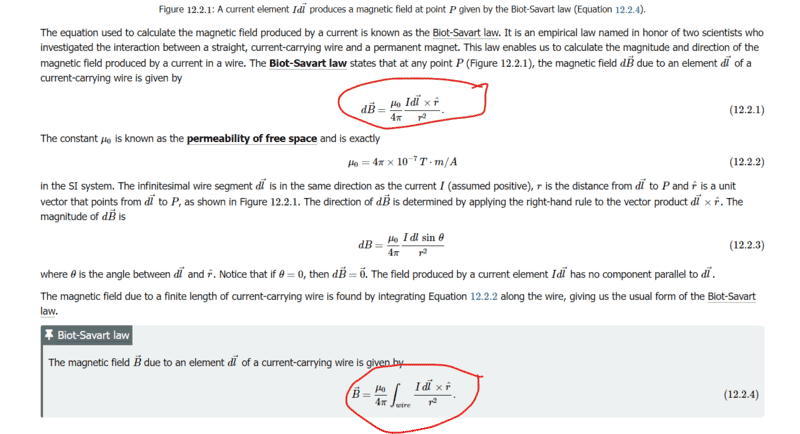Would the lower limit be 0 and the upper limit be B? How would you tell?

Many thanks!

#### Attachments

Staff Emeritus
Homework Helper
Gold Member
You are integrating along the wire, not over a magnetic field.

•ChiralSuperfields
Homework Helper
Gold Member
The vector sum of all elements ##d\vec B## is, by definition, ##\vec B##. The symbolic form ##\int \! d\vec B## in plain English says "add vectorially all contributions to the magnetic field at a given point." When you do that, you get (what else?) the total field at that point which is denoted by ##\vec B##.

We've been through this before with the meaning of ##\int \! dQ## in the case of a charged ring. Think of integration as a summation where a representative piece of what you are adding is the integrand.

•ChiralSuperfields
ChiralSuperfields
You are integrating along the wire, not over a magnetic field.
Thanks for your reply @Orodruin ! Sorry what do you mean that we are no integrating over the magnetic field?

Many thanks!

ChiralSuperfields
The vector sum of all elements ##d\vec B## is, by definition, ##\vec B##. The symbolic form ##\int \! d\vec B## in plain English says "add vectorially all contributions to the magnetic field at a given point." When you do that, you get (what else?) the total field at that point which is denoted by ##\vec B##.

We've been through this before with the meaning of ##\int \! dQ## in the case of a charged ring. Think of integration as a summation where a representative piece of what you are adding is the integrand.
Thanks for your reply @kuruman ! I understand what you are saying about that the integral is the sum of each magnetic field vector. However, is it possible to have a limits of integration for the left-hand side. It appears if use the lower limit of integration to be B and the upper limit to be zero I get their correct left-hand side.

Many thanks!

Homework Helper
Gold Member
However, is it possible to have a limits of integration for the left-hand side. It appears if use the lower limit of integration to be B and the upper limit to be zero I get their correct left-hand side.
I don't know what you mean about the correct left-hand side using upper and lower limits. ##\vec B## is a vector, not a function of some variable that has values over an interval.

A statement in English (no symbols)
When I add (superimpose) all magnetic field contributions at a given point in space from various segments of the wire, I get a vector that is the same as the total magnetic field at that point.

The same statement in mathematese (no English)
##\int d\vec B=\vec B.##

•ChiralSuperfields
ChiralSuperfields
I don't know what you mean about the correct left-hand side using upper and lower limits. ##\vec B## is a vector, not a function of some variable that has values over an interval.

A statement in English (no symbols)
When I add (superimpose) all magnetic field contributions at a given point in space from various segments of the wire, I get a vector that is the same as the total magnetic field at that point.

The same statement in mathematese (no English)
##\int d\vec B=\vec B.##
Thanks for your reply @kuruman ! Are you saying that we cannot have limits of integration like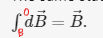even though it gives the correct result?

Why would we not be able to have limits of integration for a vector? Do we have to split it up into sperate integrals then combine, for example: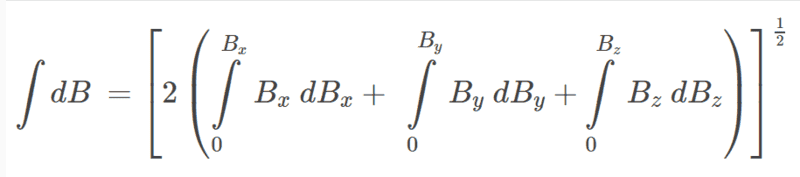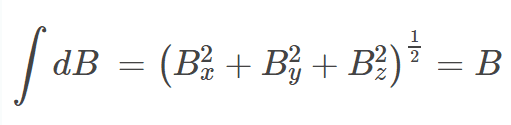(Where B is the magnetic field vector)

Many thanks,
Callum

#### Attachments

Homework Helper
Gold Member
No, no, no. You cannot convert a vector into a scalar.

##d\vec B## is a vector and has three components.
##d\vec B=dB_x~\mathbf{\hat x} +dB_y~\mathbf{\hat y}+dB_z~\mathbf{\hat z}~## and
##\int d\vec B=\int dB_x~\mathbf{\hat x} +\int dB_y~\mathbf{\hat y}+\int dB_z~\mathbf{\hat z}.##

That's how you add vectors, isn't it? How much formal instruction in calculus have you had?

•ChiralSuperfields
ChiralSuperfields
No, no, no. You cannot convert a vector into a scalar.

##d\vec B## is a vector and has three components.
##d\vec B=dB_x~\mathbf{\hat x} +dB_y~\mathbf{\hat y}+dB_z~\mathbf{\hat z}~## and
##\int d\vec B=\int dB_x~\mathbf{\hat x} +\int dB_y~\mathbf{\hat y}+\int dB_z~\mathbf{\hat z}.##

That's how you add vectors, isn't it? How much formal instruction in calculus have you had?
Thanks for your reply @kuruman ! Not too much, I have not done multivariate or vector calculus.

Do you please know why I can't convert the vector into a scalar?

Kind regards,
Callum

Homework Helper
Gold Member
Thanks for your reply @kuruman ! Not too much, I have not done multivariate calculus.

Kind regards,
Callum
Then I recommend that you take it at your earliest opportunity. It will pull things together for you.

•ChiralSuperfields
ChiralSuperfields
##d\vec B## is a vector and has three components.
##d\vec B=dB_x~\mathbf{\hat x} +dB_y~\mathbf{\hat y}+dB_z~\mathbf{\hat z}~## and
##\int d\vec B=\int dB_x~\mathbf{\hat x} +\int dB_y~\mathbf{\hat y}+\int dB_z~\mathbf{\hat z}.##
Thanks for your help @kuruman ! Why are there no limits of integration for each integral component of the magnetic field vector?

Many thanks,
Callum

ChiralSuperfields
Then I recommend that you take it at your earliest opportunity. It will pull things together for you.
Ok thank you @kuruman, I might be able to take a course later next year.

ChiralSuperfields
Then I recommend that you take it at your earliest opportunity. It will pull things together for you.
Hi @kuruman,

I looked into some vector calculus about integrating vector valued functions. I read that the integral of a vector valued function (the magnetic field vector in this case) is the integral of each component. however, now I would like to understand why there are no limits of integration for the RHS (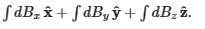).

I decided to add some and found that the limits below seem to work: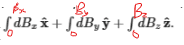The textbook I'm using makes no mention why they did not include limits of integration and no mention of vector calculus. I guess the reason why they may not have included limits of integration is because they are summing all the small magnetic fields dB, but I would like to know the physical meaning of the limits of integration in this case.

Many thanks,
Callum

Last edited:
Staff Emeritus
Homework Helper
Gold Member
That it gives the correct result does not mean that it ”works”. Even if you insert boundaries like that it is meaningless as you do not know hat the components are. The integration domain is subtextual and universally understood to be the sum over all contributions to the magnetic field. You are looking for a particular further meaning where there is none.

•ChiralSuperfields
ChiralSuperfields
That it gives the correct result does not mean that it ”works”. Even if you insert boundaries like that it is meaningless as you do not know what the components are. The integration domain is subtextual and universally understood to be the sum over all contributions to the magnetic field. You are looking for a particular further meaning where there is none.
Ok thank you @Orodruin !

Homework Helper
Gold Member
Hi @kuruman,

I looked into some vector calculus about integrating vector valued functions. I read that the integral of a vector valued function (the magnetic field vector in this case) is the integral of each component. however, now I would like to understand why there are no limits of integration for the RHS (View attachment 318817).

I decided to add some and found that the limits below seem to work:
View attachment 318818

The textbook I'm using makes no mention why they did not include limits of integration and no mention of vector calculus. I guess the reason why they may not have included limits of integration is because they are summing all the small magnetic fields dB, but I would like to know the physical meaning of the limits of integration in this case.

Many thanks,
Callum
You are asking me for the physical meaning of the upper and lower limits to the integrals ##\int dB_x##, etc. I think I answered that in post #6. Let me first solve a simple problem where upper and lower have physical meaning.

A car is moving at constant acceleration ##a=4.0~##m/s2. At ##t=3.0~##s its velocity is ##-2.0~## m/s. Find its velocity at ##t=5.0~##s.

You can solve this in your head. If the velocity of the car increases by ##4.0## m/s for every second that goes by, then from ##3.0## s to ##5.0## seconds, it will increase by ##8.0## m/s and will be ##-2+8=6~##m/s.

But the point is not to get the right answer but to illustrate the physical meaning of limits of integration. We start with the basic equation ##dv=a~dt## and integrate both sides. What limits of integration should we choose? Note that
(a) on the LHS we have dimensions of velocity and on the RHS we have dimensions of time.
(b) the lower and upper limits in the velocity must match the lower and upper limits in the time interval
(c) the upper limit that corresponds to the velocity at time ##t=5.0## s is unknown so we call it ##v.##

Integrating both sides, we write \begin{align} & \int_{-2.0~\text{m/s}}^v dv=\int_{3.0~\text{s}}^{5.0~\text{s}}4~(\text{m}/\text{s}^2)dt \nonumber \\ & v-(-2.0~\text{m/s})=4~(\text{m}/\text{s}^2)(5.0~\text{s}-3.0~\text{s}) \nonumber \\ & v+2.0~\text{m/s}=20.0~\text{m/s}-12.0~\text{m/s} \nonumber \\ & v=6.0~\text{m/s}. \nonumber \end{align}Do you see the physical significance of the upper and lower limits and how they are arranged to match on the two sides? I chose non-zero lower limits to indicate to you that the lower limits is not zero unless there is a physical reason to be so.

The only physical meaning that the lower and upper limits of ##0## and ##B_x## that I can see is that zero is what you have for the sum before you start adding the x-components and ##B_x## is what you end up with when you're done adding. However, that is true for any sum regardless of what's on the RHS.

I have nothing more to say on this. If you still have questions, perhaps someone else will be able to answer them better than me.

•ChiralSuperfields
ChiralSuperfields
You are asking me for the physical meaning of the upper and lower limits to the integrals ##\int dB_x##, etc. I think I answered that in post #6. Let me first solve a simple problem where upper and lower have physical meaning.

A car is moving at constant acceleration ##a=4.0~##m/s2. At ##t=3.0~##s its velocity is ##-2.0~## m/s. Find its velocity at ##t=5.0~##s.

You can solve this in your head. If the velocity of the car increases by ##4.0## m/s for every second that goes by, then from ##3.0## s to ##5.0## seconds, it will increase by ##8.0## m/s and will be ##-2+8=6~##m/s.

But the point is not to get the right answer but to illustrate the physical meaning of limits of integration. We start with the basic equation ##dv=a~dt## and integrate both sides. What limits of integration should we choose? Note that
(a) on the LHS we have dimensions of velocity and on the RHS we have dimensions of time.
(b) the lower and upper limits in the velocity must match the lower and upper limits in the time interval
(c) the upper limit that corresponds to the velocity at time ##t=5.0## s is unknown so we call it ##v.##

Integrating both sides, we write \begin{align} & \int_{-2.0~\text{m/s}}^v dv=\int_{3.0~\text{s}}^{5.0~\text{s}}4~(\text{m}/\text{s}^2)dt \nonumber \\ & v-(-2.0~\text{m/s})=4~(\text{m}/\text{s}^2)(5.0~\text{s}-3.0~\text{s}) \nonumber \\ & v+2.0~\text{m/s}=20.0~\text{m/s}-12.0~\text{m/s} \nonumber \\ & v=6.0~\text{m/s}. \nonumber \end{align}Do you see the physical significance of the upper and lower limits and how they are arranged to match on the two sides? I chose non-zero lower limits to indicate to you that the lower limits is not zero unless there is a physical reason to be so.

The only physical meaning that the lower and upper limits of ##0## and ##B_x## that I can see is that zero is what you have for the sum before you start adding the x-components and ##B_x## is what you end up with when you're done adding. However, that is true for any sum regardless of what's on the RHS.

I have nothing more to say on this. If you still have questions, perhaps someone else will be able to answer them better than me.
Thanks for your help @kuruman ! I think you are making sense about the physical meaning you described:To me that makes sense since when you are starting summing x-component then there will be no component initially however, eventually across the wire the x-components will sum to the resultant x-component of the magnetic field which is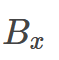.

However, I tried to have a look graphically but was not too sure what to put on the y-axis.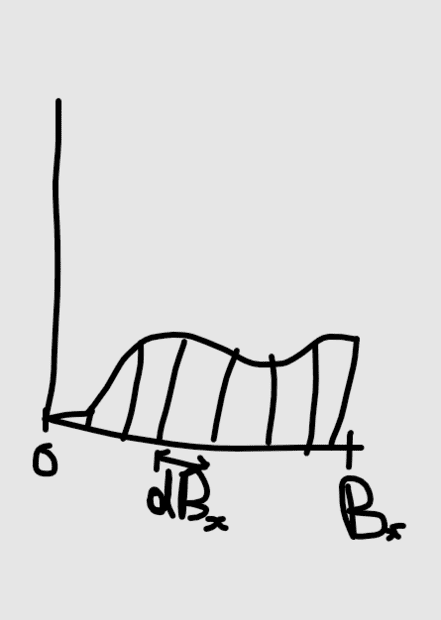Many thanks,
Callum

Homework Helper
Gold Member
However, I tried to have a look graphically but was not too sure what to put on the y-axis
You are not sure because there is nothing that can go on the y-axis. I explained the principle at work several times before but you don't seem to get it. I will try one last time with an analogy and that will be it for me.

Consider a whole Genoa salami that is ##x=50~##cm long. Cut it up into ##N## pieces so that their thicknesses are ##dx_1,~dx_2,~dx_3,~\dots dx_N##.

Question: What do you get when you put the pieces back together again?
Answer: You get a Genoa salami that is 50 cm long.
Question: If each slice were subdivided into many more pieces, would the answer above change?

Followup questions
Question 2: What about if you do the same to a stick of pepperoni that is ##x=60~##cm long?
Answer 2: You get a stick of pepperoni that is 60 cm long and the number of slices doesn't matter.

Question 3: What about if you do the same to a board of wood that is ##x=6~##ft long?
Answer 3: You get a board of wood that is 6 ft long and the number of slices doesn't matter.

Question 4: What about if you do the same to a magnetic field component that is ##B_x~##Tesla long?
Answer 4: You get a ________ that is _____ Tesla long and the number of slices doesn't matter.
(Fill in the blanks)

What conclusions do you draw from all this?

N.B. The same principle would also work with Humpty Dumpty if only all the king's horses and all the king's men could put him back together again.

•ChiralSuperfields
ChiralSuperfields
You are not sure because there is nothing that can go on the y-axis. I explained the principle at work several times before but you don't seem to get it. I will try one last time with an analogy and that will be it for me.

Consider a whole Genoa salami that is ##x=50~##cm long. Cut it up into ##N## pieces so that their thicknesses are ##dx_1,~dx_2,~dx_3,~\dots dx_N##.

Question: What do you get when you put the pieces back together again?
Answer: You get a Genoa salami that is 50 cm long.
Question: If each slice were subdivided into many more pieces, would the answer above change?

Followup questions
Question 2: What about if you do the same to a stick of pepperoni that is ##x=60~##cm long?
Answer 2: You get a stick of pepperoni that is 60 cm long and the number of slices doesn't matter.

Question 3: What about if you do the same to a board of wood that is ##x=6~##ft long?
Answer 3: You get a board of wood that is 6 ft long and the number of slices doesn't matter.

Question 4: What about if you do the same to a magnetic field component that is ##B_x~##Tesla long?
Answer 4: You get a ________ that is _____ Tesla long and the number of slices doesn't matter.
(Fill in the blanks)

What conclusions do you draw from all this?

N.B. The same principle would also work with Humpty Dumpty if only all the king's horses and all the king's men could put him back together again.